﻿ Free fall and heaping of discrete particles - XSim

# Free fall and heaping of discrete particles

Update: March 18, 2018
OpenFOAM 4.x

## Case directory

\$FOAM_TUTORIALS/lagrangian/MPPICFoam/column

## Summary

We calculate the free fall and heaping of discrete particles in a rectangular space, taking into account the particle volume.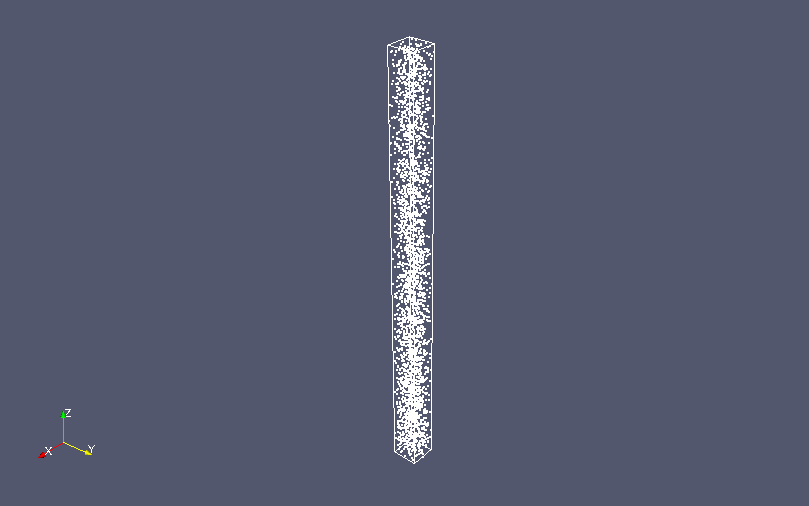Model geometry (Analysis domain and discrete particles)

The initial positions and properties of the discrete particles are defined in the files "kinematicCloudPositions" and "kinematicCloudProperties" in the directory "constant", respectively.

The meshes are as follows.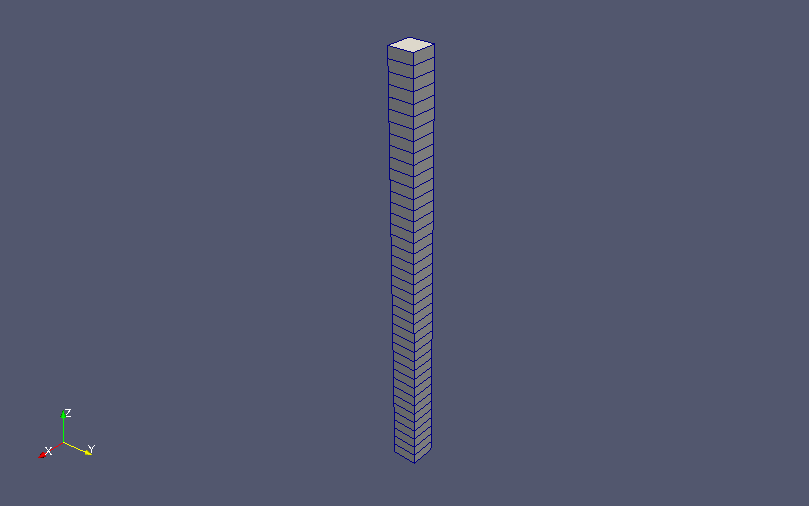Meshes

The calculation result is as follows.

Discrete particles's motion (After the start of particle inflow, the color represents velocity)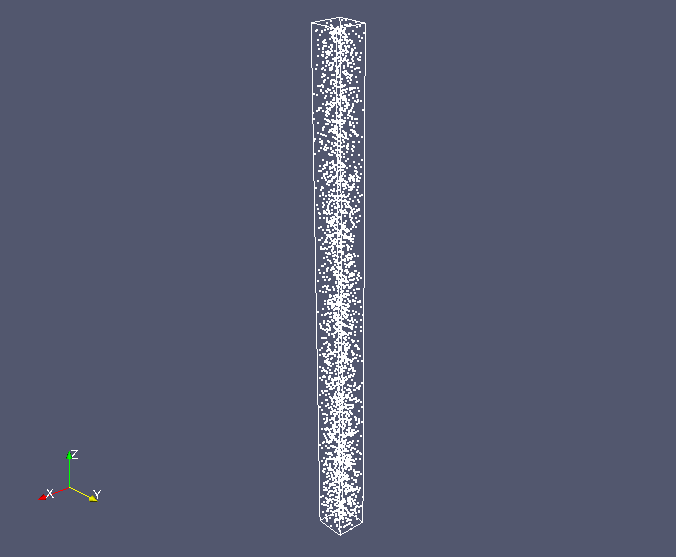Discrete particles at 0.01 sec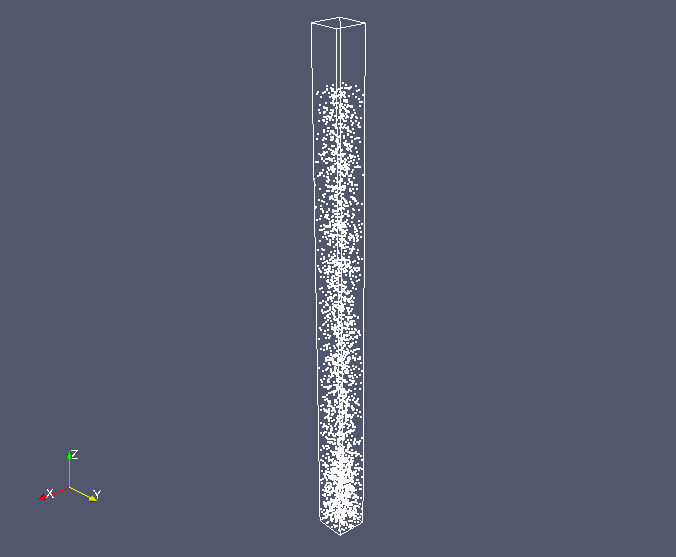Discrete particles at 0.1 sec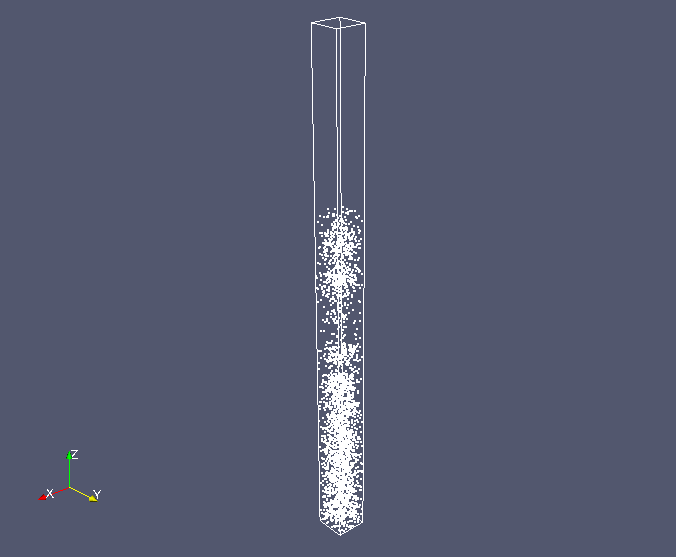Discrete particles at 0.2 sec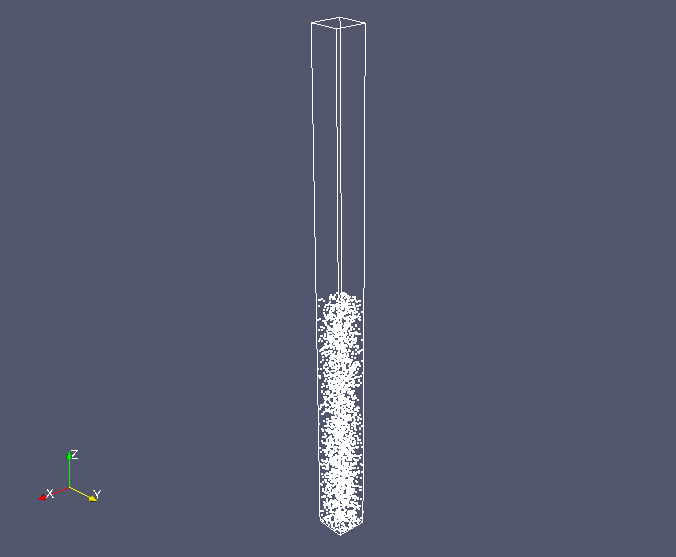Discrete particles at 1.0 sec

At around 0.2 seconds, the particle seems to be stuck in the middle of the calculation region, but the pressure distribution shows that the overall pressure is oscillating, so the calculation may become unstable state.

## Commands

cp -r \$FOAM_TUTORIALS/lagrangian/MPPICFoam/column column
cd column

blockMesh
MPPICFoam

paraFoam

## Calculation time

3 minutes 15.81 seconds *Single, Inter(R) Core(TM) i7-2600 CPU @ 3.40GHz 3.40GHz